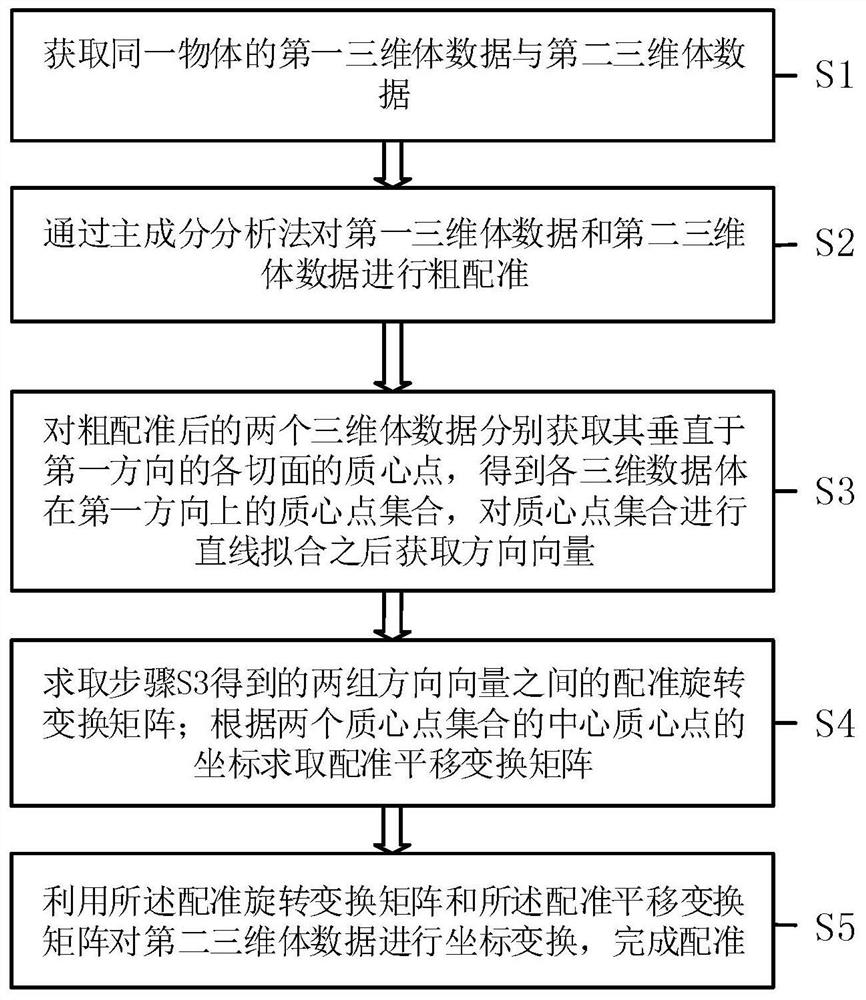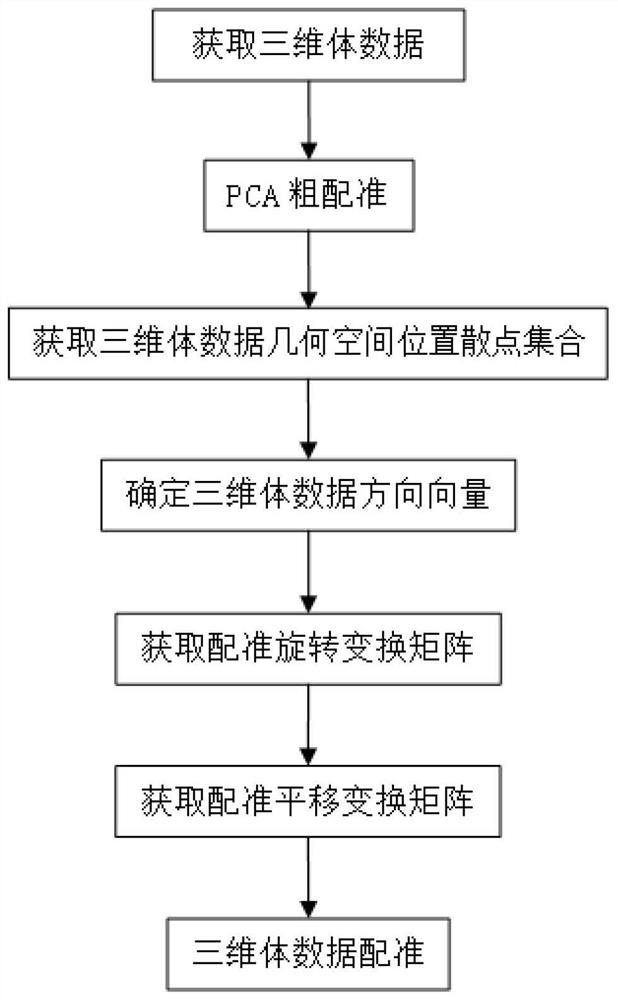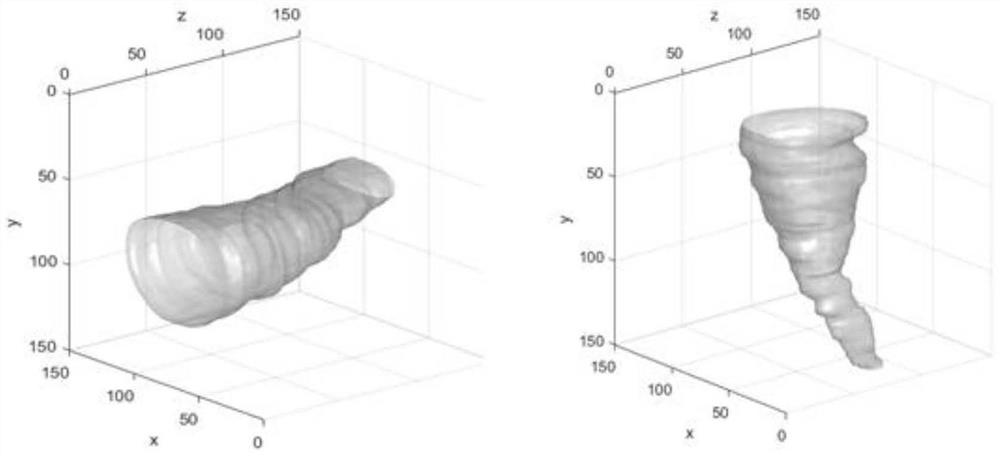# Spatial registration method and device for three-dimensional volume data

## A spatial registration and three-dimensional body technology, applied in image data processing, character and pattern recognition, image analysis, etc., can solve the problems of no simplified point set, long matching process, slow matching speed, etc., to improve registration efficiency The effect of real-time performance, shortened registration time, and reduced size

Pending Publication Date: 2022-07-29

0 Cites 0 Cited by

## AI-Extracted Technical Summary

### Problems solved by technology

 The purpose of the present invention is to overcome the problems in the prior art that the matching process takes a long time and the matchin...
View more

## Abstract

The invention relates to the field of computer vision and image processing, in particular to a spatial registration method and device for three-dimensional volume data, and the method comprises the steps: S1, obtaining first and second three-dimensional volume data of the same object; s2, performing coarse registration on the first three-dimensional body data and the second three-dimensional body data through a principal component analysis method; s3, centroid points of all tangent planes, perpendicular to the first direction, of the two pieces of three-dimensional volume data obtained after coarse registration are obtained, a centroid point set of all the three-dimensional volume data is obtained, and after straight line fitting is conducted on the set, a direction vector is obtained; s4, solving a registration rotation transformation matrix between the two groups of direction vectors; solving a registration translation transformation matrix according to the coordinates of the center centroid points of the two centroid point sets; and S5, performing coordinate transformation on the second three-dimensional volume data by using the registration rotation transformation matrix and the registration translation transformation matrix to complete registration. According to the method, the geometric space information of the three-dimensional volume data is fully utilized, the data set size is reduced, the registration time is shortened, and the registration efficiency and real-time performance are improved.

Application Domain

Image enhancementImage analysis +1

Technology Topic

Point setComputer vision and image processing +7

## Image

•••## Examples

• Experimental program(1)

### Example Embodiment

 Example 1
 This embodiment takes the registration of carotid blood vessel data as an example, and provides a spatial registration method for three-dimensional volume data, such as figure 1 shown, including the following steps:
 S1, obtaining the first three-dimensional volume data and the second three-dimensional volume data of the same object;
 S2, performing rough registration on the first three-dimensional volume data and the second three-dimensional volume data by principal component analysis;
 S3: Obtain the centroid points of each section perpendicular to the first direction for the two three-dimensional volume data after the rough registration, obtain the centroid point set of each three-dimensional volume data in the first direction, and perform straight line fitting on the centroid point set. After the combination, the direction vector is obtained;
 S4, obtain the registration rotation transformation matrix between the two groups of direction vectors obtained in step S3; obtain the registration translation transformation matrix according to the coordinates of the center centroid points of the two centroid point sets;
 S5 , performing coordinate transformation on the second three-dimensional volume data by using the registration rotation transformation matrix and the registration translation transformation matrix to complete the registration.
 In this embodiment, taking the Z-axis direction as the first direction, step S3 and step S4 are further decomposed, and the following steps are provided: figure 2 Detailed steps shown:
 Step 1. Acquire 3D volume data.
 The original 3D volume data Vo and the target 3D volume data Vt to be registered are obtained. The two sets of 3D volume data have the same spatial pixel distance and the same size, and are 3D volume data of the same object acquired in different periods or under different conditions. The original 3D volume data Vo such as image 3 As shown in the left figure of , the target 3D volume data Vt to be registered is as follows image 3 shown on the right.
 Step 2. PCA rough registration.
 The original three-dimensional volume data Vo and the target three-dimensional volume data Vt to be registered are processed by the classical PCA method to obtain the first principal component direction vector of the two, which are (x o ,y o ,z o ) and (x t ,y t ,z t ),as follows Figure 4 shown, Figure 4 The left picture is the first principal component direction vector of the original 3D volume data Vo, Figure 4 The figure on the right shows the first principal component direction vector of the target 3D volume data Vt to be registered. Rotate the original 3D volume data Vo and the target 3D volume data Vt to be registered to be parallel to the Z axis of the coordinate system according to the first principal component direction vector to obtain the coarsely registered 3D volume data Vo' (corresponding to the original 3D volume data Vo) and the three-dimensional volume data Vt' (corresponding to the target three-dimensional volume data Vt to be registered), taking this coordinate system as the spatial coordinate system of the three-dimensional volume data, the three-dimensional volume data Vo' and Vt' after rough registration are respectively as follows Figure 5 shown on the left and right.
 Step 3: Obtain a set of scattered points of geometric space positions of the three-dimensional volume data.
 After rough registration of the three-dimensional volume data Vo' and Vt', the geometric centroid points of all XY cuts perpendicular to the Z-axis direction are obtained respectively. The specific method is to record the coordinate of each pixel on the XY slice in the X direction as x i , the coordinate in the Y direction is y i , the corresponding pixel value is p i , then the coordinate x of the centroid in the X direction and the coordinate y in the Y direction are:

 In the Z direction, each centroid point on the XY section forms a scatter point set under the three-dimensional coordinate system containing the geometric space position information of the three-dimensional volume data, that is, the centroid point set, and the obtained centroid point coordinates are normalized. In this step, the obtained three-dimensional volume data Vo' and the three-dimensional volume data Vt' are respectively obtained as follows: Image 6 shown on the left and right.
 Step 4: Determine the direction vector of the three-dimensional volume data.
 According to the set of three-dimensional centroid points of the three-dimensional volume data Vo' and the three-dimensional volume data Vt' obtained in step 3, a three-dimensional straight line is fitted, and the direction vector (x) of the fitted straight line is obtained respectively. o ',y o ',z o ') and (x t ',y t ',z t '), respectively representing the spatial direction information of the 3D volume data Vo' and the 3D volume data Vt', respectively Figure 7 shown on the left and right.
 Step 5. Obtain the registration rotation transformation matrix.
 According to the two sets of direction vectors (x o ',y o ',z o ') and (x t ',y t ',z t '), calculate the direction vector (x t ',y t ',z t ') and the direction vector (x o ',y o ',z o ') The angle that needs to be rotated to remain parallel. The rotation angle θ around the X-axis can be obtained by the dot product and cross product of the vector x :
 θ x =atan(((0,y t ',z t ')*(0,y o ',z o '))/((0,y t ',z t ')×(0,y o ',z o '))),
 Further, obtain the X-direction rotation matrix M x :

 Similarly, the rotation angle θ around the Y-axis can be obtained y and the rotation angle θ around the Z axis z , Y and Z direction rotation matrix M y and M z :
 θ y =atan(((x t ',0,z t ')*(x o ',0,z o '))/((x t ',0,z t ')×(x o ',0,z o ')))
 θ z =atan(((x t ',y t ',0)*(x o ',y o ',0))/((x t ',y t ',0)×(x o ',y o ',0)))


 Thus, the registration rotation transformation matrix M is obtained r =M z *M y *M x.
 Step 6: Obtain a registration translation transformation matrix.
 According to the set of centroid points of the three-dimensional volume data Vo' obtained in step 3, the center centroid point C is obtained o =(x 1 , y 1 ,z 1 ), in the same way, the center centroid point C of the three-dimensional volume data Vt' can be obtained t =(x 2 , y 2 ,z 2 ), find the translation coordinate C=C 0 -C t =(x 1 -x 2 , y 1 -y 2 ,z 1 -z 2 ) to obtain the registration translation transformation matrix M s =[x 1 -x 2 y 1 -y 2 z 1 -z 2 ].
 Step 7, 3D volume data registration.
 According to the registration and rotation transformation matrix M obtained in step 5 and step 6 r with the registration translation transformation matrix M s , perform coordinate transformation on the three-dimensional volume data Vt', so that it is transformed into the same coordinate system as the three-dimensional volume data Vo', complete the registration of the three-dimensional volume data, and obtain the registered three-dimensional volume data V r , the original 3D volume data and the registered 3D volume data are as follows Figure 8 shown on the left and right.
In addition to taking the Z-axis direction as the first direction in this embodiment, based on the same principle, in other embodiments, the Y-axis direction can also be used as the first direction to acquire all XZ directions of the three-dimensional volume data perpendicular to the Y-axis direction The geometric centroid point on the slice; or, taking the X-axis direction as the first direction, obtain the geometric centroid point of the 3D volume data on all YZ slices perpendicular to the X-axis direction.
 According to the three-dimensional volume data of the target to be registered and the original three-dimensional volume data, the method provided by the invention uses the centroid point of the two-dimensional section to simplify the three-dimensional volume data set, reduces the three-dimensional registration problem to two-dimensional to solve, and calculates the to-be-registered The direction vector and translation coordinates of the target 3D volume data are registered, the rotation transformation matrix and the translation transformation matrix are registered, and the 3D volume data is registered and transformed. The invention makes full use of the geometric space information of the three-dimensional volume data, reduces the size of the data set, shortens the registration time, and improves the registration efficiency and real-time performance.
 Although this embodiment takes the registration of the three-dimensional volume data of the carotid artery as an example, the method provided by the present invention can also be used for other parts of the human body or animals, medical equipment and other general object surface geometry in the field of medical imaging In addition, in addition to the field of medical imaging, the commonly used fields of the method and device of the present invention include reverse engineering, industrial inspection, computer vision, CG production, 3D printing and intelligent manufacturing, etc. involving computer vision and image processing technical field.

## PUM## Description & Claims & Application Information

We can also present the details of the Description, Claims and Application information to help users get a comprehensive understanding of the technical details of the patent, such as background art, summary of invention, brief description of drawings, description of embodiments, and other original content. On the other hand, users can also determine the specific scope of protection of the technology through the list of claims; as well as understand the changes in the life cycle of the technology with the presentation of the patent timeline. Login to view more.

## Business realization method and device for programmable devices and communication terminal

ActiveCN107544819Areduce sizeSave configuration space
Owner:ZTE CORP

## Configuration resource near-field transmission method and device

InactiveCN106375461Areduce sizeshorten the time
Owner:LETV HLDG BEIJING CO LTD +1

## A character string construction method and device in game application

InactiveCN109885396Areduce sizeGuaranteed game frame rate
Owner:ZHUHAI KINGSOFT ONLINE GAME TECH CO LTD +1

## Control method for single-phase off-network inverter power supply

InactiveCN106849725AStrong resistance to shock loadsreduce size
Owner:GUANGXI POWER GRID CO LTD NANNING POWER SUPPLY BUREAU

## A hook framework technology based on an apk file of an Android application

Owner:SUNINFO INFORMATION TECH

• reduce size

## Embedded type system storage space optimization method

ActiveCN101187899Areduce sizeReduce storage media capacity
Owner:ZTE CORP

## TFT array substrate manufacture method

ActiveCN105702623Areduce sizeincrease pixel density
Owner:WUHAN CHINA STAR OPTOELECTRONICS TECH CO LTD

## Audio file cutting method and device and terminal equipment

InactiveCN106935253Areduce sizeImprove the level of utilization efficiency
Owner:BEIJING ANYUNSHIJI TECH CO LTD

## Power supply power distribution system and method

InactiveCN103532402Areduce sizeReduce the cross-sectional area
Owner:SICHUAN INJET ELECTRIC CO LTD

## Arc-control device of breaker

ActiveCN102945768ASmall operating powerreduce size
Owner:CHINA XD ELECTRIC CO LTD +1
Who we serve
• R&D Engineer
• R&D Manager
• IP Professional
Why Eureka